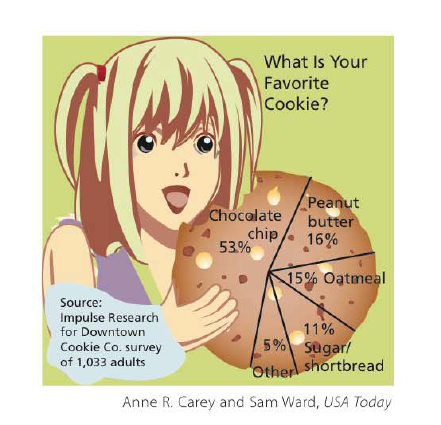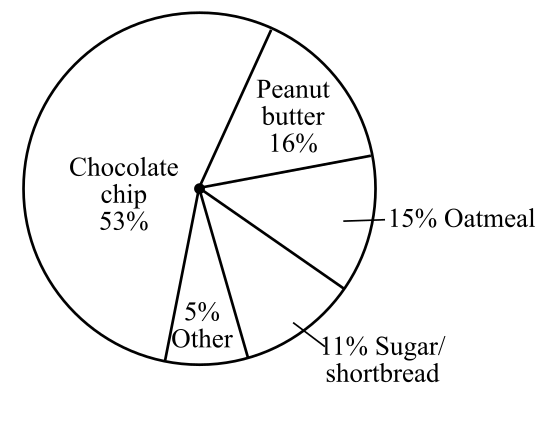Chapter 6.I, Problem 45RE### Contemporary Mathematics for Busin...

8th Edition
Robert Brechner + 1 other
ISBN: 9781305585447

#### Solutions

Chapter
Section### Contemporary Mathematics for Busin...

8th Edition
Robert Brechner + 1 other
ISBN: 9781305585447
Textbook Problem

# Use the pie chart “What is Your Favorite Cookie?” to find the decimal and reduced fraction equivalent for exercises 41–45. Type of Cookie Decimal Reduced Fraction 45. OtherTo determine

To calculate: The decimal and reduced fraction value of the other cookies by using the following pie chart.Explanation

Given Information:

The provided pie chart is,

Formula used:

Follow the steps as mentioned below to convert percentage value into decimal value.

Step1: Take away the percentage sign.

Step2: Divide the value obtained in step 1 by 100.

Follow the steps as mentioned below to convert percentage value into reduced fraction value.

Step1: Take away the percentage sign.

Step2: Now put the number with the value of percent as the numerator and 100 as the denominator, then reduce the fraction to its lowest term.

Calculation:

From the provided pie chart the value of other cookies is 5%.

Compute the decimal value of the other cookies as follows:

Take away the percentage sign,

5

Divide by 100 and simplify,

5100=0

### Still sussing out bartleby?

Check out a sample textbook solution.

See a sample solution

#### The Solution to Your Study Problems

Bartleby provides explanations to thousands of textbook problems written by our experts, many with advanced degrees!

Get Started

#### f(x)=12x+2

Precalculus: Mathematics for Calculus (Standalone Book)

#### Define sampling with replacement and explain why is it used?

Statistics for The Behavioral Sciences (MindTap Course List)

#### The angle θ at the right has cosine:

Study Guide for Stewart's Multivariable Calculus, 8th

#### What is a ceiling effect, and how can it be a problem?

Research Methods for the Behavioral Sciences (MindTap Course List)